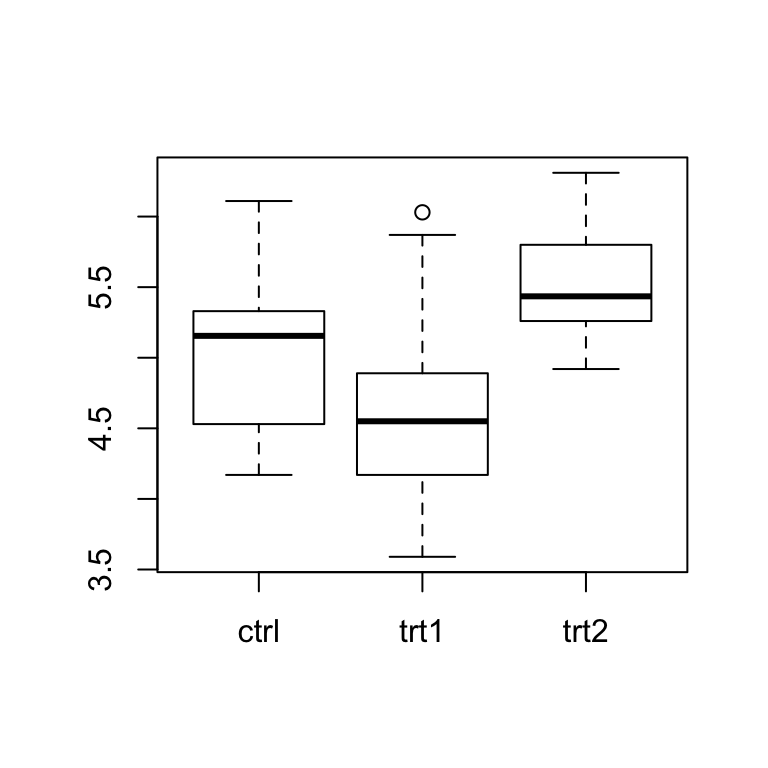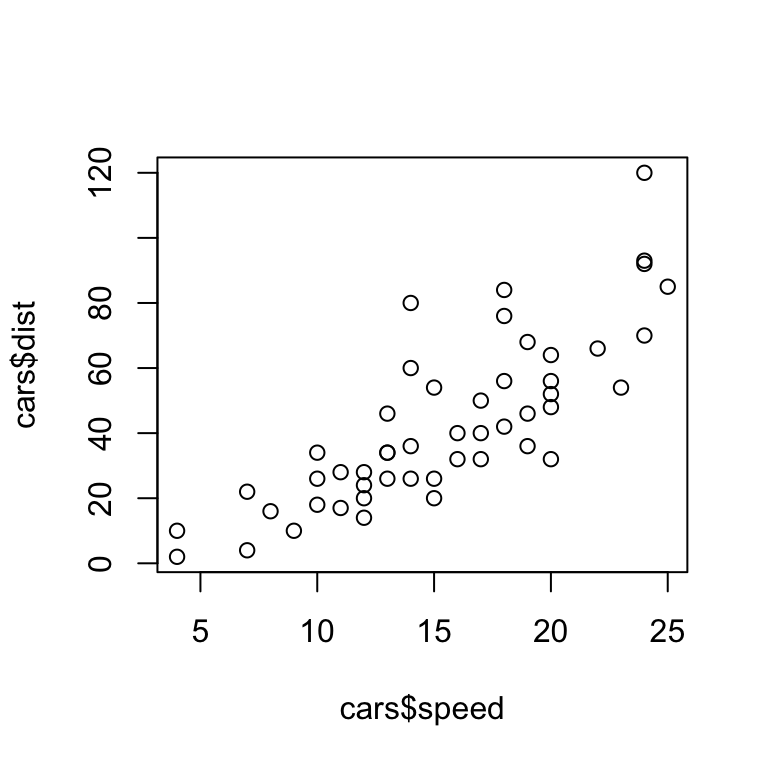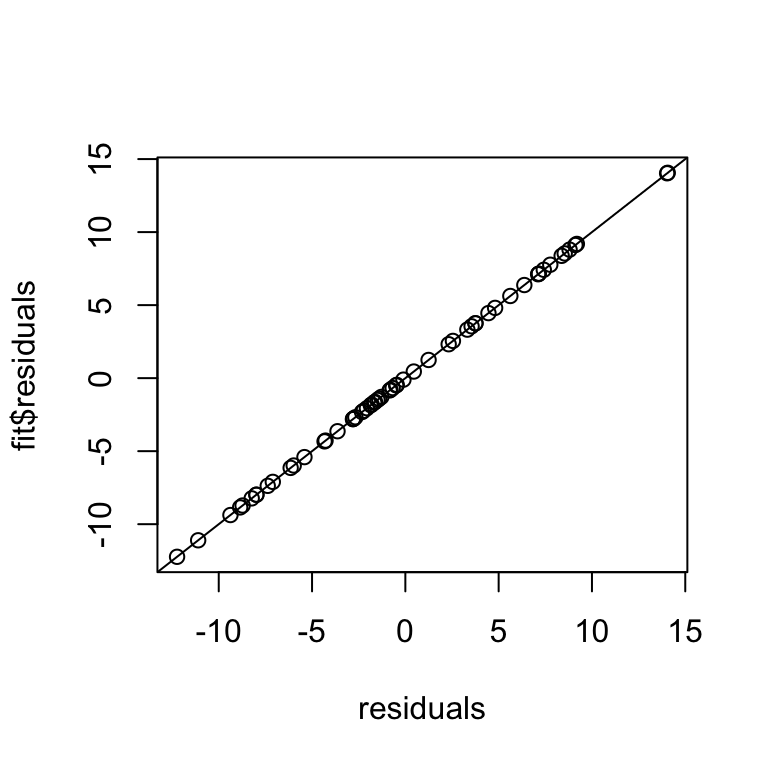Jan 24, 2019

## 1. t test

We will try the t test on the built-in data set PlantGrowth. However, first we need to reformat the data set, which we do with the function unstack(). We store the reformatted data set in a variable plants:

head(PlantGrowth)
##   weight group
## 1   4.17  ctrl
## 2   5.58  ctrl
## 3   5.18  ctrl
## 4   6.11  ctrl
## 5   4.50  ctrl
## 6   4.61  ctrl
plants <- unstack(PlantGrowth)
head(plants)
##   ctrl trt1 trt2
## 1 4.17 4.81 6.31
## 2 5.58 4.17 5.12
## 3 5.18 4.41 5.54
## 4 6.11 3.59 5.50
## 5 4.50 5.87 5.37
## 6 4.61 3.83 5.29

The data set contains plant growth yield (dry weight) under one control and two treatment conditions:

boxplot(plants)Question: Is the mean control weight significantly different from the mean weight under treatment 1? Is the mean weight under treatment 1 significantly different from the mean weight under treatment 2? Use the function t.test() to find out.

First, control vs. treatment 1:

t.test(plants$ctrl, plants$trt1)
##
##  Welch Two Sample t-test
##
## data:  plants$ctrl and plants$trt1
## t = 1.1913, df = 16.524, p-value = 0.2504
## alternative hypothesis: true difference in means is not equal to 0
## 95 percent confidence interval:
##  -0.2875162  1.0295162
## sample estimates:
## mean of x mean of y
##     5.032     4.661

The p-value is 0.25. We cannot reject H0. Control and treatment 1 do not appear to be different.

Second, treatment 1 vs. treatment 2:

t.test(plants$trt1, plants$trt2)
##
##  Welch Two Sample t-test
##
## data:  plants$trt1 and plants$trt2
## t = -3.0101, df = 14.104, p-value = 0.009298
## alternative hypothesis: true difference in means is not equal to 0
## 95 percent confidence interval:
##  -1.4809144 -0.2490856
## sample estimates:
## mean of x mean of y
##     4.661     5.526

The p-value is 0.009. We reject H0. Plants seem to grow more under treatment 2 than under treatment 1.

## 2. Correlation

We will try the correlation test on the built-in data set cars. The data set contains the speed of cars and the distances taken to stop, measured in the 1920s:

head(cars)
##   speed dist
## 1     4    2
## 2     4   10
## 3     7    4
## 4     7   22
## 5     8   16
## 6     9   10

Is there a relationship between speed and stopping distance? Use the function cor.test() to find out. Then make a scatterplot of speed vs. stopping distance, using the function plot().

cor.test(cars$speed, cars$dist)
##
##  Pearson's product-moment correlation
##
## data:  cars$speed and cars$dist
## t = 9.464, df = 48, p-value = 1.49e-12
## alternative hypothesis: true correlation is not equal to 0
## 95 percent confidence interval:
##  0.6816422 0.8862036
## sample estimates:
##       cor
## 0.8068949
plot(cars$speed, cars$dist)There is a significant positive relationship between a car’s speed and its stopping distance. The correlation coefficient is 0.81, i.e., 66% of the variation in a car’s stopping distance is explained by the car’s speed. (Remember, the square of the correlation coefficient, i.e. here 0.81^2=0.66, tells us the amount of variation explained by the correlation.)

## 3. Regression

We will do a regression analysis on the data set cabbages from the R package MASS. The data set contains the weight (HeadWt), vitamin C content (VitC), the cultivar (Cult), and the planting date (Date) for 60 cabbage heads:

library(MASS) # load the MASS library to make the data set available
head(cabbages)
##   Cult Date HeadWt VitC
## 1  c39  d16    2.5   51
## 2  c39  d16    2.2   55
## 3  c39  d16    3.1   45
## 4  c39  d16    4.3   42
## 5  c39  d16    2.5   53
## 6  c39  d16    4.3   50

Use a multivariate regression to find out whether weight and cultivar have an effect on the vitamin C content. You will need to use the functions lm() and summary().

To run a linear regression, you need to first fit the model to the data. This is done with the function lm() (lm stands for Linear Model). The lm() function takes two arguments, the formula (here VitC ~ Cult + HeadWt) and the data (here cabbages). The formula describes what kind of model we want to fit. On the left-hand side of the symbol ~, we write the response variable, here VitC. On the right-hand side, we write the predictor variables we want to use, separated by a + sign. Here, we use Cult and HeadWt as predictor variables. (You can learn more about formulas in R by typing ?formula into the R console.)

fit <- lm(VitC ~ Cult + HeadWt, data=cabbages)

Once you have run the linear model, you can then display the results using the summary() function:

summary(fit)
##
## Call:
## lm(formula = VitC ~ Cult + HeadWt, data = cabbages)
##
## Residuals:
##     Min      1Q  Median      3Q     Max
## -12.233  -3.796  -1.065   4.542  14.061
##
## Coefficients:
##             Estimate Std. Error t value Pr(>|t|)
## (Intercept)  67.9297     3.1159  21.801  < 2e-16 ***
## Cultc52       9.3578     1.7433   5.368 1.52e-06 ***
## HeadWt       -5.6524     0.9962  -5.674 4.88e-07 ***
## ---
## Signif. codes:  0 '***' 0.001 '**' 0.01 '*' 0.05 '.' 0.1 ' ' 1
##
## Residual standard error: 6.304 on 57 degrees of freedom
## Multiple R-squared:  0.625,  Adjusted R-squared:  0.6119
## F-statistic:  47.5 on 2 and 57 DF,  p-value: 7.234e-13

We see that both the cultivar and the weight have a significant effect on vitamin C content. The negative estimate for HeadWt indicates that as the weight increases, vitamin C content decreases.

Often, the function anova() provides a simpler and cleaner summary of the model fit:

anova(fit)
## Analysis of Variance Table
##
## Response: VitC
##           Df Sum Sq Mean Sq F value    Pr(>F)
## Cult       1 2496.2 2496.15  62.811 9.145e-11 ***
## HeadWt     1 1279.5 1279.48  32.196 4.884e-07 ***
## Residuals 57 2265.2   39.74
## ---
## Signif. codes:  0 '***' 0.001 '**' 0.01 '*' 0.05 '.' 0.1 ' ' 1

The conclusions remain unchanged. Both the cultivar and the weight have a significant effect on vitamin C content.

## 4. If this was easy

Look into the function predict(). Can you use it to estimate the vitamin C content of a c52 cultivar with a weight of 4? Can you use it to calculate the residuals of the regression model?

The predict function allows us to predict values from a linear model that we have previously fit. It takes two arguments, the fitted model (fit from the previous subsection) and a data frame that has the same columns as were used as predictor variables in the linear model. Thus, to estimate the vitamin C content of a c52 cultivar with a weight of 4, we first create a data frame with one row. Then we run predict():

d <- data.frame(Cult="c52", HeadWt=4) # make a data frame with 1 row
predict(fit, d) # run predict on previously fitted model with new data frame
##        1
## 54.67786

We predict that the vitamin C content of a c52 cultivar with a weight of 4 is 54.7.

If we run predict with the original data frame then we get the model estimate for each data point (these values are also called the fitted values). By subtracting these values from the original y values we obtain the residuals:

residuals <- cabbages$VitC - predict(fit, cabbages) Note that the residuals are also available as fit$residuals. The following plot shows that the two sets of numbers are identical:

plot(residuals, fit\$residuals)
abline(0, 1) # add one-one line